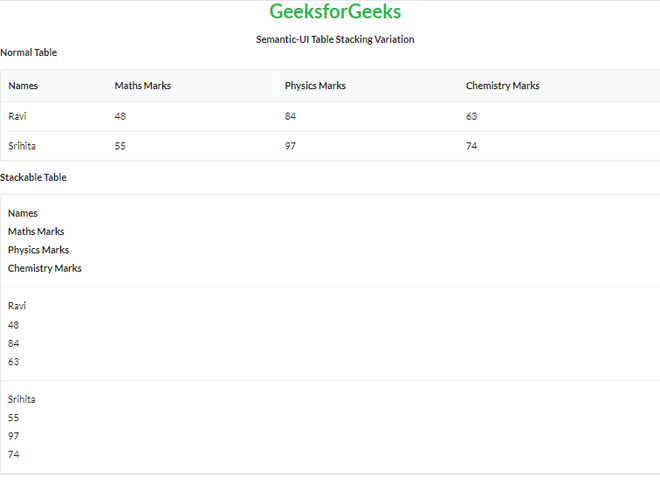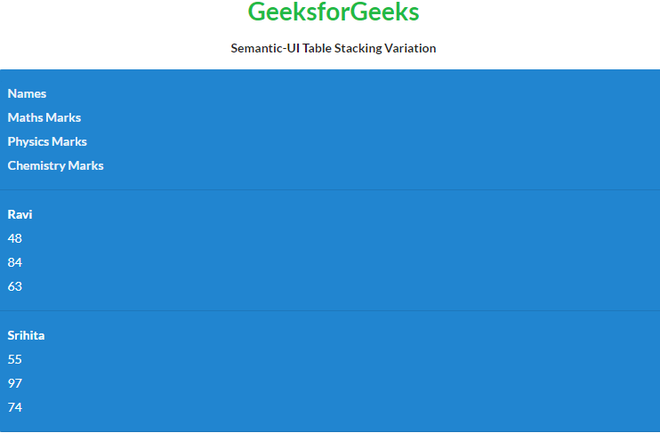Open in App
Not now

# Semantic-UI Table Stacking Variation

• Last Updated : 17 Mar, 2022

Semantic UI is an open-source framework that uses CSS and jQuery to build great user interfaces. It is the same as a bootstrap for use and has great different elements to use to make your website look more amazing. It uses a class to add CSS to the elements.

Tables are an easy way to organize a lot of data. A table is an arrangement of data in rows and columns, or possibly in a more complex structure. Tables are widely used in communication, research, and data analysis. Tables are useful for various tasks such as presenting text information and numerical data. It can be used to compare two or more items in the tabular form layout. Tables are used to create databases. An HTML table and a Semantic UI table both are the same structurally.

Table stacking variation of Semantic UI specifies how that table stacks table content responsively.

Semantic UI Table Stacking Variation Classes:

• stackable: This class stacks the table responsively.
• unstackable: This class divides each row of our table into separate cells.

Syntax:

```<table class="ui stackable table">
<tr>
<td></td>
...
</tr>
...
</table>```

Example 1: This is a basic example illustrating the difference between a normal unstackable table and a stackable table on tablet size devices created using Semantic UI.

## HTML

 ` ` `<``html``> ` `<``head``> ` `    ``<``title``>Semantic-UI Table Stacking Variation ` `    ``<``link` `href``= ` `"https://cdnjs.cloudflare.com/ajax/libs/semantic-ui/2.4.1/semantic.min.css"`  `          ``rel``=``"stylesheet"` `/> ` ` ` `<``body``> ` `    ``<``center``> ` `        ``<``h1` `class``=``"ui green header"``>GeeksforGeeks ` `        ``<``strong``>Semantic-UI Table Stacking Variation ` `    `` ` `    ``<``b``>Normal Table ` `    ``<``table` `class``=``"ui unstackable table"``> ` `        ``<``thead``> ` `            ``<``tr``> ` `                ``<``th``>Names ` `                ``<``th``>Maths Marks ` `                ``<``th``>Physics Marks ` `                ``<``th``>Chemistry Marks ` `            `` ` `        `` ` `        ``<``tbody``> ` `          ``<``tr``> ` `            ``<``td``>Ravi ` `            ``<``td``>48 ` `            ``<``td``>84 ` `            ``<``td``>63 ` `          `` ` `          ``<``tr``> ` `            ``<``td``>Srihita ` `            ``<``td``>55 ` `            ``<``td``>97 ` `            ``<``td``>74 ` `          `` ` `      `` ` `    `` ` `    ``<``b``>Stackable Table ` `    ``<``table` `class``=``"ui tablet stackable table"``> ` `        ``<``thead``> ` `            ``<``tr``> ` `                ``<``th``>Names ` `                ``<``th``>Maths Marks ` `                ``<``th``>Physics Marks ` `                ``<``th``>Chemistry Marks ` `            `` ` `        `` ` `        ``<``tbody``> ` `          ``<``tr``> ` `            ``<``td``>Ravi ` `            ``<``td``>48 ` `            ``<``td``>84 ` `            ``<``td``>63 ` `          `` ` `          ``<``tr``> ` `            ``<``td``>Srihita ` `            ``<``td``>55 ` `            ``<``td``>97 ` `            ``<``td``>74 ` `          `` ` `      `` ` `    `` ` ` ` ``

Output:Semantic-UI Table Stacking Variation

Example 2: This is a basic example illustrating colored (inverted) stackable table variation on tablet size devices created using Semantic UI.

## HTML

 ` ` `<``html``> ` `<``head``> ` `    ``<``title``>Semantic-UI Table Stacking Variation ` `    ``<``link` `href``= ` `"https://cdnjs.cloudflare.com/ajax/libs/semantic-ui/2.4.1/semantic.min.css"`  `         ``rel``=``"stylesheet"` `/>        ` ` ` `<``body``> ` `    ``<``center``> ` `        ``<``h1` `class``=``"ui green header"``>GeeksforGeeks ` `        ``<``strong``>Semantic-UI Table Stacking Variation ` `    `` ` `    ``<``table` `class``=``"ui tablet inverted blue stackable table"``> ` `        ``<``thead``> ` `            ``<``tr``> ` `                ``<``th``>Names ` `                ``<``th``>Maths Marks ` `                ``<``th``>Physics Marks ` `                ``<``th``>Chemistry Marks ` `            `` ` `        `` ` `        ``<``tbody``> ` `          ``<``tr``> ` `            ``<``td``>Ravi ` `            ``<``td``>48 ` `            ``<``td``>84 ` `            ``<``td``>63 ` `          `` ` `          ``<``tr``> ` `            ``<``td``>Srihita ` `            ``<``td``>55 ` `            ``<``td``>97 ` `            ``<``td``>74 ` `          `` ` `      `` ` `    `` ` ` ` ``

Output:Semantic-UI Table Stacking Variation

My Personal Notes arrow_drop_up
Related Articles